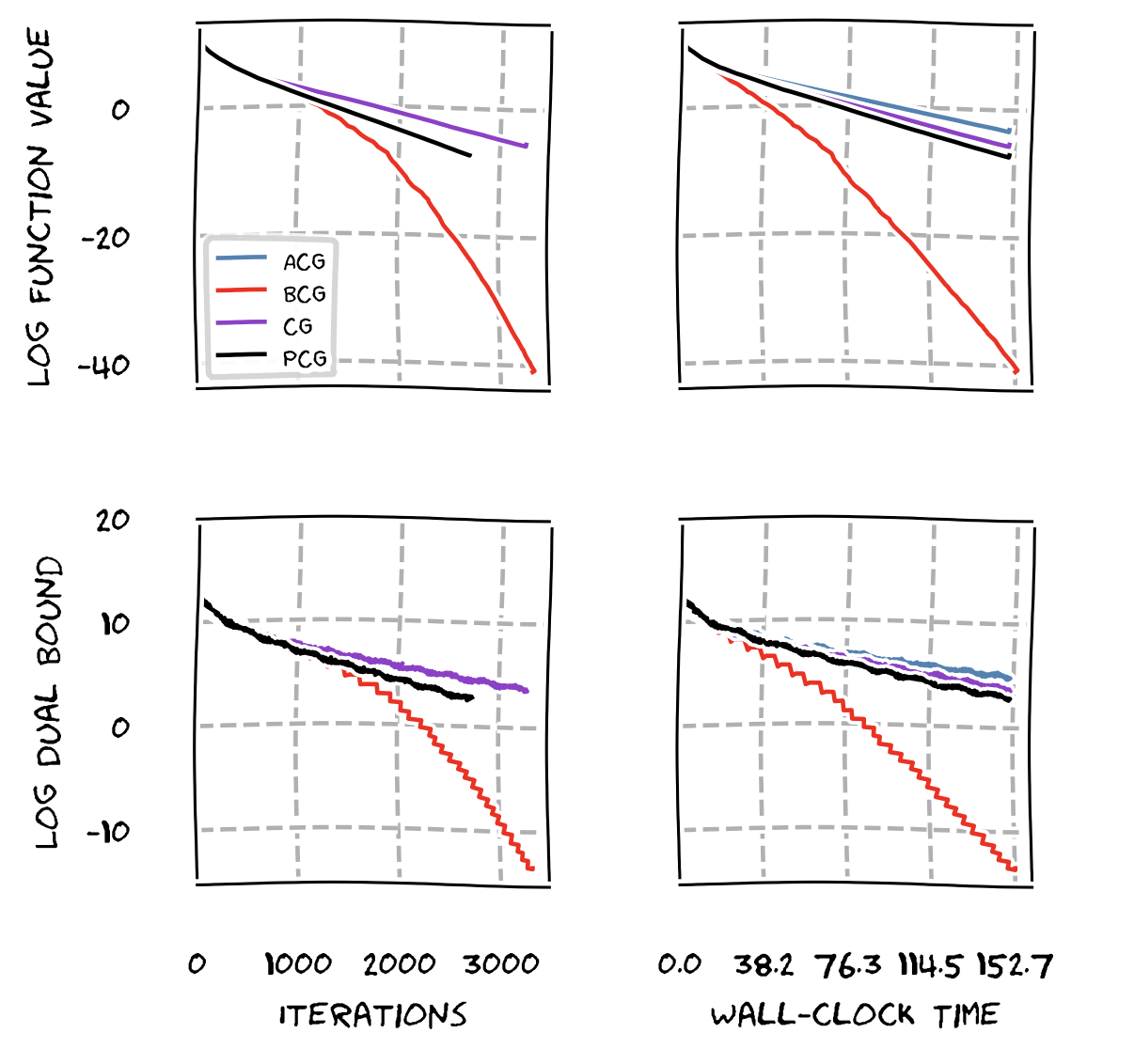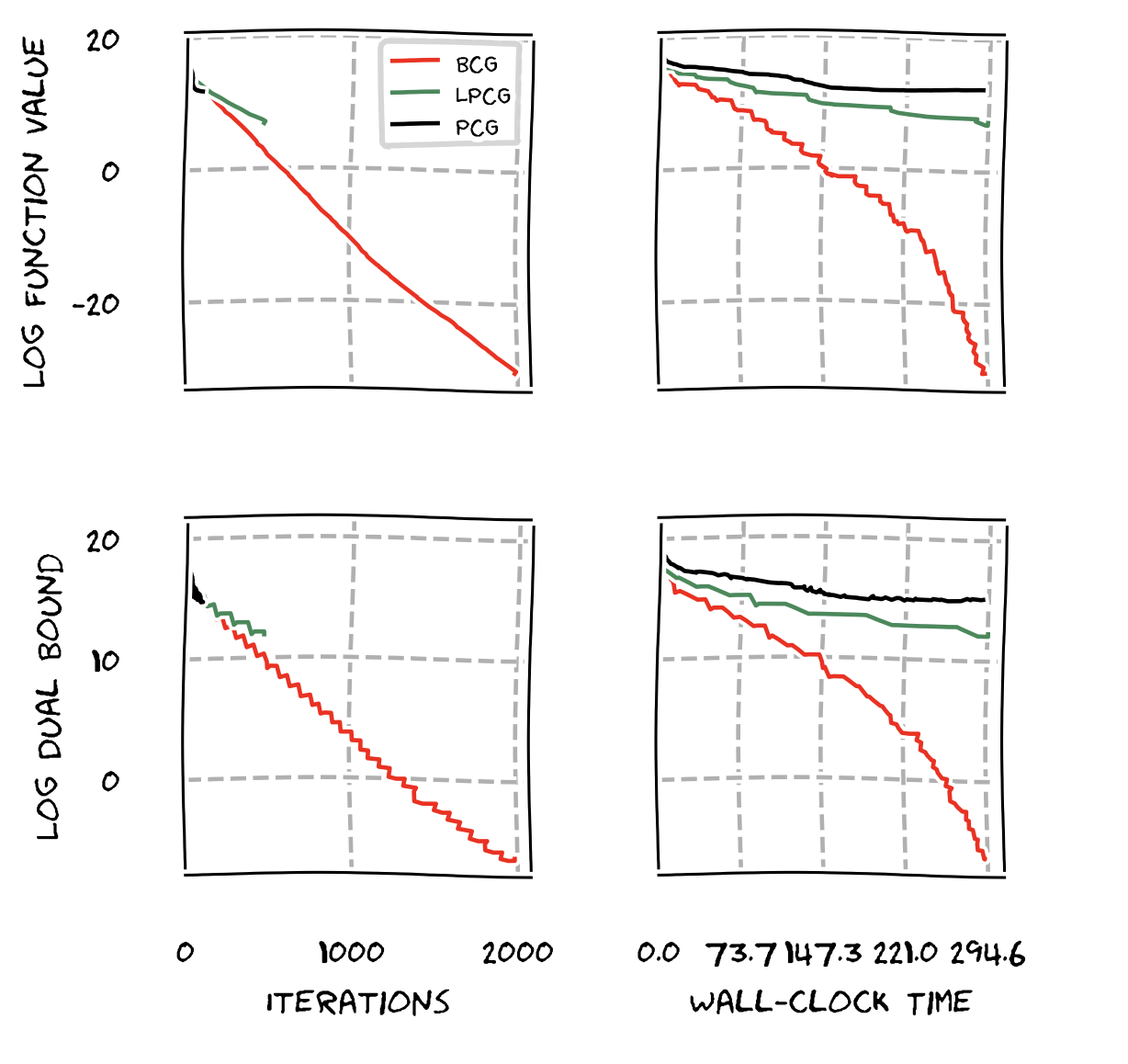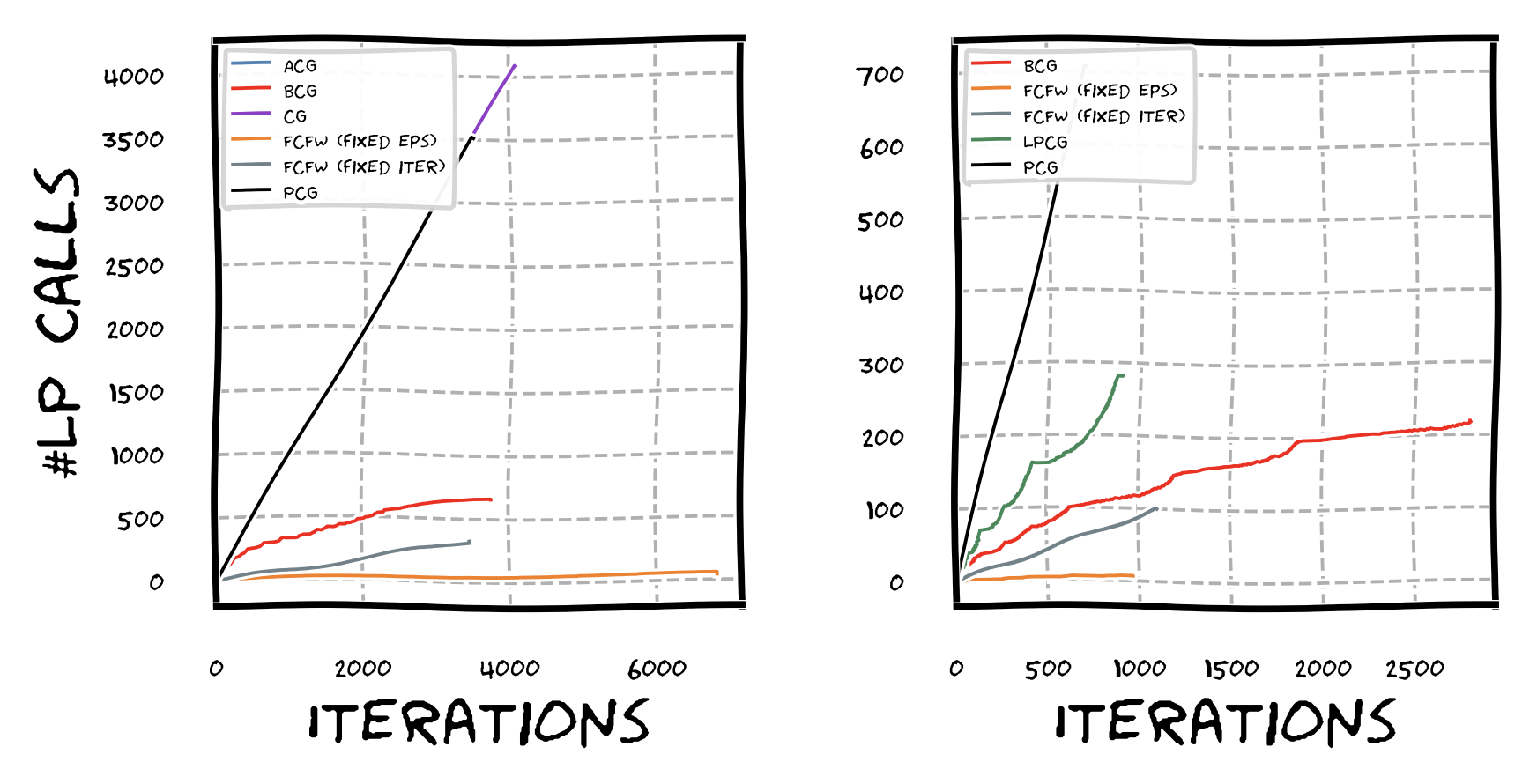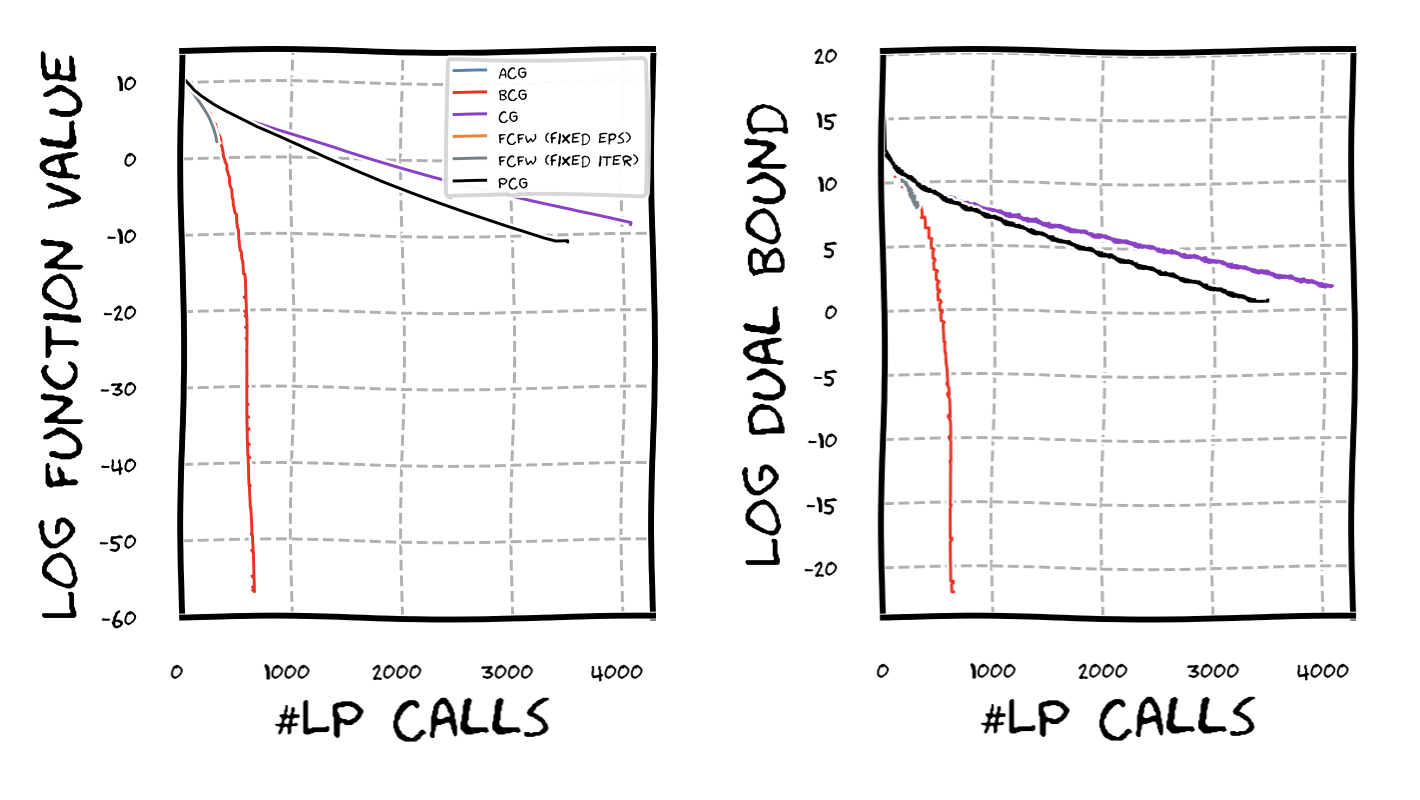TL;DR: This is an informal summary of our recent paper Blended Conditional Gradients with Gábor Braun, Dan Tu, and Stephen Wright, showing how mixing Frank-Wolfe and Gradient Descent gives a new, very fast, projection-free algorithm for constrained smooth convex minimization.

## What is the paper about and why you might care

Frank-Wolfe methods [FW] (also called conditional gradient methods [CG]) have been very successful in solving constrained smooth convex minimization problems of the form:

$\min_{x \in P} f(x),$

where $P$ is some compact and convex feasible region; you might want to think of, e.g., $P$ being a polytope, which is one of the most common cases. We assume so-called first-order access to the objective function $f$, i.e., we have an oracle that returns function evaluation $f(x)$ and gradient information $\nabla f(x)$ for a provided point $x \in P$. Moreover, we assume that we have access to the feasible region $P$ by means of a so-called linear optimization oracle, which upon being presented with a linear objective $c \in \RR^n$ returns $\arg\min_{x \in P} \langle c, x \rangle$. The basic Frank-Wolfe algorithm looks like this:

Frank-Wolfe Algorithm [FW]
Input: Smooth convex function $f$ with first-order oracle access, feasible region $P$ with linear optimization oracle access, initial point (usually a vertex) $x_0 \in P$.
Output: Sequence of points $x_0, \dots, x_T$
For $t = 1, \dots, T$ do:
$\quad v_t \leftarrow \arg\min_{x \in P} \langle \nabla f(x_{t-1}), x \rangle$
$\quad x_{t+1} \leftarrow (1-\gamma_t) x_t + \gamma_t v_t$

The Frank-Wolfe algorithm has a couple of important advantages:

1. It is very easy to implement
2. It does not require projections (as projected gradient descent does)
3. It maintains iterates as reasonably sparse convex combination of vertices.

Generally, one can expect an $O(1/t)$ convergence for the general convex smooth case and linear convergence for strongly convex functions with appropriate modifications of the Frank-Wolfe algorithm. The interested reader might check out Cheat Sheet: Frank-Wolfe and Conditional Gradients and Cheat Sheet: Linear convergence for Conditional Gradients for an extensive overview.

In the context of Frank-Wolfe methods a key assumption is that linear optimization is cheap. Compared to projections one would have to perform, say for projected gradient descent this is almost always true (except for very simple feasible regions where projection is trivial). As such traditionally one would account for the linear optimization oracle call with an $O(1)$ cost and disregard it in the analysis. However, if the feasible region is complex (e.g., arising from an integer program or just being a really large linear program), this assumption is not warranted anymore and one might ask a few natural questions:

1. Do we really have to call the (expensive) linear programming oracle in each iteration?
2. Do we really need to compute (approximately) optimal solutions to the LP or does something completely different suffice?
3. More generally, can we reuse information?

It turns out that one can replace the linear programming oracle by what we call a weak separation oracle; see [BPZ] for more details. Without going into full detail, as I will have a dedicated post about lazification (what we dubbed this technique), what the oracle does, it basically wraps around the actual linear programming oracle. Before calling the linear programming oracle, the weak separation oracle can answer by checking previous answers to oracle calls (caching). Moreover, one gains that one does not have to solve the LPs to (approximate) optimality but rather it suffices to check for a certain minimal improvement, which is compatible with the to-be-achieved convergence rate. In particular, we do not need any optimality proofs. One can then show that one can maintain the same convergence rates using the weak separation oracle as for the respective Frank-Wolfe variant utilizing the linear programming oracle, while drastically reducing the number of LP oracle calls.

## Our results

In practice, while lazification can provide huge speedups for Frank-Wolfe type methods when the LPs are hard to solve, this technique loses its advantage when the LPs are simple. The reason for this is that at the end of the day, there is a trade-off between the quality of the computed directions in terms of providing progress vs. how hard they are to compute: the weak-separation oracle computes potentially worse approximations but does so very fast.

However, what we show in our Blended Conditional Gradients paper is that one can:

1. Cut-out a huge fraction of LP oracle calls (sometimes less than 1% of the iterations require an actual LP oracle call)
2. While working with actual gradients as descent direction providing much better progress than traditional Frank-Wolfe directions and
3. Staying fully projection-free.

This is achieved by blending together conditional gradient descent steps and gradient steps in a special way. The resulting algorithm has a per-iteration cost that is very comparable to gradient descent in most of the steps and when the LP oracle is called the per-iteration cost is comparable to the standard Frank-Wolfe Algorithm. In progress per iteration though our algorithm, which we call Blended Conditional Gradients (BCG) (see [BPTW] for details), typically outperforms Away-Step and Pairwise Conditional Gradients (the current state-of-the-art methods). We are often even faster in wall-clock performance as we eschew most LP oracle calls. Naturally, we maintain worst-case convergence rates that match those of Away-Step Frank-Wolfe and Pairwise Conditional Gradients; the known lower bounds only assume first-order oracle access and LP oracle access and are unconditional.

Rather than stating the algorithm’s (worst-case) convergence rates for the various cases which are identical to the ones for Away-Step Frank-Wolfe and Pairwise Conditional Gradients achieving $O(1/\varepsilon)$-convergence for general smooth and convex functions and $O(\log 1/\varepsilon)$-convergence for smooth and strongly convex functions (see [LJ] and [BPTW] for details), I rather present some computational results as they highlight the typical behavior. The following graphics provide a pretty representative overview of the computational performance. Everything is in log-scale and we ran each algorithm with a fixed time limit; we refer the reader to [BPTW] for more details.

The first example is a benchmark of BCG vs. Away-Step Frank-Wolfe (AFW), Pairwise Conditional Gradients (PCG), and Vanilla Frank-Wolfe (FW) on a LASSO instance. BCG significantly outperforms the other variants and in fact the empirical convergence rate of BCG is much higher than the rates of the other algorithms; recall we are in log-scale and we expect linear convergence for all variants due to the characteristics of the instance (optimal solution in strict interior).One might say that the above is not completely unexpected in particular because BCG uses also the lazification technique from our previous work in [BPZ]. So let us see how we compare to lazified variants of Frank-Wolfe. In the next graph we compare BCG vs. LPCG vs. PCG. The problem we solve here is a structured regression problem over a spanning tree polytope. Clearly, while LPCG is faster than PCG, BCG is significantly faster than either of those, both in iterations and wall-clock time.To better understand what is going on let us see how often the LP oracle is actually called throughout the iterations. In the next graph we plot iterations vs. cumulative number of calls to the (true) LP oracle. Here we added also Fully-Corrective Frank-Wolfe (FCFW) variants that fully optimize out over the active set and hence should have the lowest number of required LP calls; we implemented two variants: one that optimizes over the active set for a fixed number of iterations (the faster one in grey) and one that optimizes to a specific accuracy (the slower one in orange). The next plot shows two instances: LASSO (left) and structured regression over a netgen instance (right); for the former lazification is not helpful as the LP oracle is too simple for the latter it is. As expected for the non-lazy variants such as FW, AFW, and PCG we have a straight line as we perform one LP call per iteration. For LCG, BCG, and the two FCFW variants we obtain a significant reduction in actual calls to the LP oracle with BCG sitting right between the (non-)lazy variants and the FCFW variants. BCG attains a large fraction of the reduction in calls of the much slower FCFW variants while being extremely fast compared to FCFW and all other variants.On a fundamental level one might argue that what it really comes down to is how well the algorithm uses the information obtained from an LP call. Clearly, there is a trade-off: on the one hand, better utilization of that information will be increasingly more expensive making the algorithm slower, so that it might be advantageous to rather do another LP call, on the other hand, calling the LP oracle too often results in suboptimal use of the LP call’s information and these calls can be expensive. Managing this tradeoff is critical to achieve high performance and BCG uses a convergence criterion to maintain a very favorable balance. To get an idea how well the various algorithms are using the LP call information, consider the next graphic, where we run various algorithms on a LASSO instance. As can be seen in primal and dual progress, BCG is using LP information in a much more aggressive way, while maintaining a very high speed—the two FCFW variants that would use the information from the LP calls even more aggressively only performed a handful of iterations though as they are extremely slow (see the grey and orange line right at the beginning of the red line). As a measure we depict primal and dual progress vs (true) LP oracle call.### BCG Code

If you are interested in using BCG, we made a preliminary version of our code available on github; a significant update with more options and additional algorithms is coming soon.

[FW] Frank, M., & Wolfe, P. (1956). An algorithm for quadratic programming. Naval research logistics quarterly, 3(1‐2), 95-110. pdf

[CG] Levitin, E. S., & Polyak, B. T. (1966). Constrained minimization methods. Zhurnal Vychislitel’noi Matematiki i Matematicheskoi Fiziki, 6(5), 787-823. pdf

[BPZ] Braun, G., Pokutta, S., & Zink, D. (2017, August). Lazifying conditional gradient algorithms. In Proceedings of the 34th International Conference on Machine Learning-Volume 70 (pp. 566-575). JMLR. org. pdf

[BPTW] Braun, G., Pokutta, S., Tu, D., & Wright, S. (2018). Blended Conditional Gradients: the unconditioning of conditional gradients. arXiv preprint arXiv:1805.07311. pdf

[LJ] Lacoste-Julien, S., & Jaggi, M. (2015). On the global linear convergence of Frank-Wolfe optimization variants. In Advances in Neural Information Processing Systems (pp. 496-504). pdf